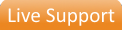LS-DYNA Validated High Speed Tensile Rate Dependent Model (MAT_019 or MAT_024 or MAT_089)

G-771V

Description

Tensile experiments are carried out over a range of strain rates ranging into the high speed impact regime. Tests include three stress-strain measurements in the .01-1 /s strain rate range and two high speed tensile stress-strain curves in the 10-100 /s range covering a total strain rate range of five decades. Poisson's ratio is measured and density is also measured. Using this material model, the tensile test is simulated in LS-DYNA at various strain rates. The response is compared with the actual tensile tests that were performed.

Included Tests

 1x D-010 Solid Density 2x M-204 Tensile Stress-Strain, Strength, and Modulus 2x M-232 High Speed Tensile Stress-Strain 1x M-206 Tensile Stress-Strain, Strength, Modulus and Poisson's Ratio

Options

-Very High Speed Tensile Stress-Strain test [M-235] for strain rates to 1000 /s

A MAT_019 or MAT_024 or MAT_089 model can be produced, upon request.

Samples

: 30 high speed tensile bars

[details]

Application

Validated material models increase the confidence that the material behavior is being properly represented in the simulation. In conjunction with LS-DYNA, this data can be used to simulate the behavior of components subjected to impact situations.